### IMO Shortlist 1984 problem 17

Kvaliteta:
Avg: 0,0
Težina:
Avg: 0,0
In a permutation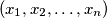$(x_1, x_2, \dots , x_n)$ of the set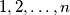$1, 2, \dots , n$ we call a pair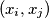$(x_i, x_j )$ discordant if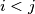$i < j$ and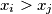$x_i > x_j$. Let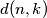$d(n, k)$ be the number of such permutations with exactly$k$ discordant pairs. Find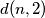$d(n, 2)$ and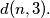$d(n, 3).$
Izvor: Međunarodna matematička olimpijada, shortlist 1984